# VisualDL Profiler Guide¶

## Introduction¶

Profiling tools is introduced in paddlepaddle since version 2.3.0. Users can collect and export profiling data of both hosts(cpu) and devices(gpu, mlu). VisualDL supports to visualize profiling data and helps you identify program bottlenecks and optimize performance. As for how to start the profiler, you can refer to doc.

## How to launch¶

1. Collect and export profiling data.

Demo:

```import paddle
import numpy as np

transform = ToTensor()
transform=transform)
transform=transform)
def __init__(self, num_classes=1):
super(MyNet, self).__init__()

self.conv1 = paddle.nn.Conv2D(in_channels=3, out_channels=32, kernel_size=(3, 3))

def forward(self, x):
x = self.conv1(x)
x = F.relu(x)
x = self.pool1(x)

x = self.conv2(x)
x = F.relu(x)
x = self.pool2(x)

x = self.conv3(x)
x = F.relu(x)

x = self.flatten(x)
x = self.linear1(x)
x = F.relu(x)
x = self.linear2(x)
return x

epoch_num = 10
batch_size = 32
learning_rate = 0.001
val_acc_history = []
val_loss_history = []

def train(model):
print('start training ... ')
# turn into training mode
model.train()

parameters=model.parameters())

shuffle=True,
batch_size=batch_size,
num_workers=1)

# create profiler
def my_on_trace_ready(prof): # callback function, will be called when profiler stops
callback = profiler.export_chrome_tracing('./profiler_demo') # export profiling data
callback(prof)
prof.summary(sorted_by=profiler.SortedKeys.GPUTotal) # print summary

p.start() # start profiler

for epoch in range(epoch_num):
x_data = data

logits = model(x_data)
loss = F.cross_entropy(logits, y_data)

if batch_id % 1000 == 0:
print("epoch: {}, batch_id: {}, loss is: {}".format(epoch, batch_id, loss.numpy()))
loss.backward()
opt.step()

p.step() # signify profiler into next step
if batch_id == 19:
p.stop() # stop profiler
exit()

# evaluate model after one epoch
model.eval()
accuracies = []
losses = []
x_data = data

logits = model(x_data)
loss = F.cross_entropy(logits, y_data)
accuracies.append(acc.numpy())
losses.append(loss.numpy())

avg_acc, avg_loss = np.mean(accuracies), np.mean(losses)
print("[validation] accuracy/loss: {}/{}".format(avg_acc, avg_loss))
val_acc_history.append(avg_acc)
val_loss_history.append(avg_loss)
model.train()

model = MyNet(num_classes=10)
train(model)
```

The code shows you how to collect profiling data in step range [3, 14) using paddle.profiler api, and export data into directory `profiler_demo`. You will see some files with extension .json in that directory.

1. Launch VisualDL to visualize data

After run above program, you can launch the panel by:

```visualdl --logdir ./profiler_demo --port 8080
```

Then, open the browser and enter the address`http://127.0.0.1:8080`, you will see the profiler panel.There are six viewers supported for your analysis, i.e. Overview, Operator, GPU Kernel, Distributed, Trace and Memory.

## Overview¶

Overview viewer illustrates a summary of model performance, consists of run configuration, device summary, execution summary, execution time breakdown, event summary, event time breakdown and user-defined summary, totally seven parts to show.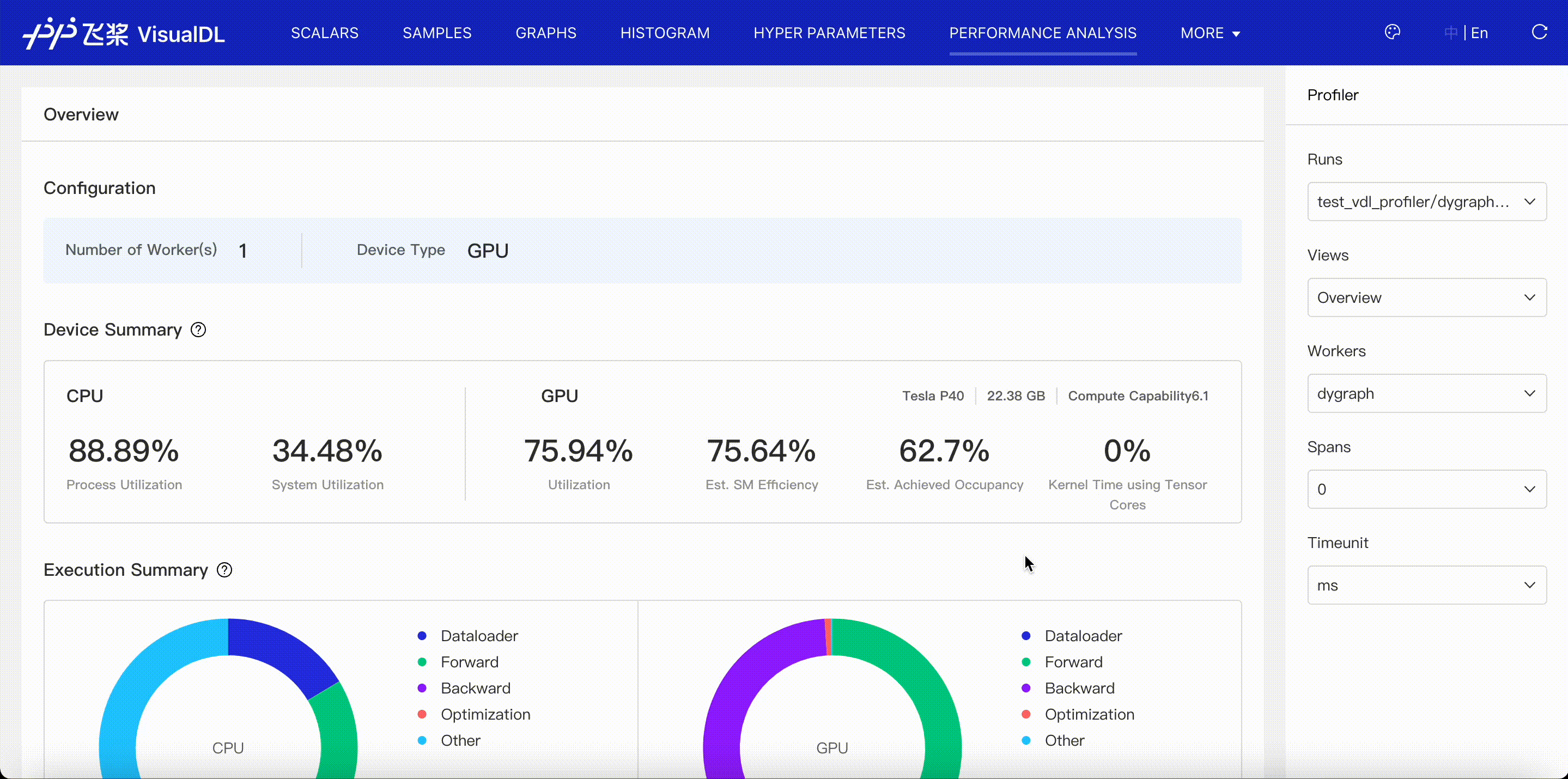• Configuration: Present all processes and their devices in current profiling data(runs).

• Device summary: Present device details, such as utilization and occupancy.

• Execution summary: Present CPU and GPU time for each stage of a model, i.e. DataLoader, Forward, Backward, Optimization and Other.

• Execution time breakdown: Present CPU and GPU time in each ProfileStep for each stage of a model, i.e. DataLoader, Forward, Backward, Optimization and Other.

• Event summary: Present the distribution of each kind of events across DataLoader, Forward, Backward, Optimization and Other stage.

• Event time breakdown: Present the time of each kind of events included in DataLoader, Forward, Backward, Optimization and Other stage.

• UserDefined summary: Present CPU and GPU time of events defined by users in python scripts.

## Operator¶

Operator viewer shows CPU and GPU time for operators in paddle framework. When you choose Operator Name + Input Shape to present the operator table, you can see operator's input shapes of tensors.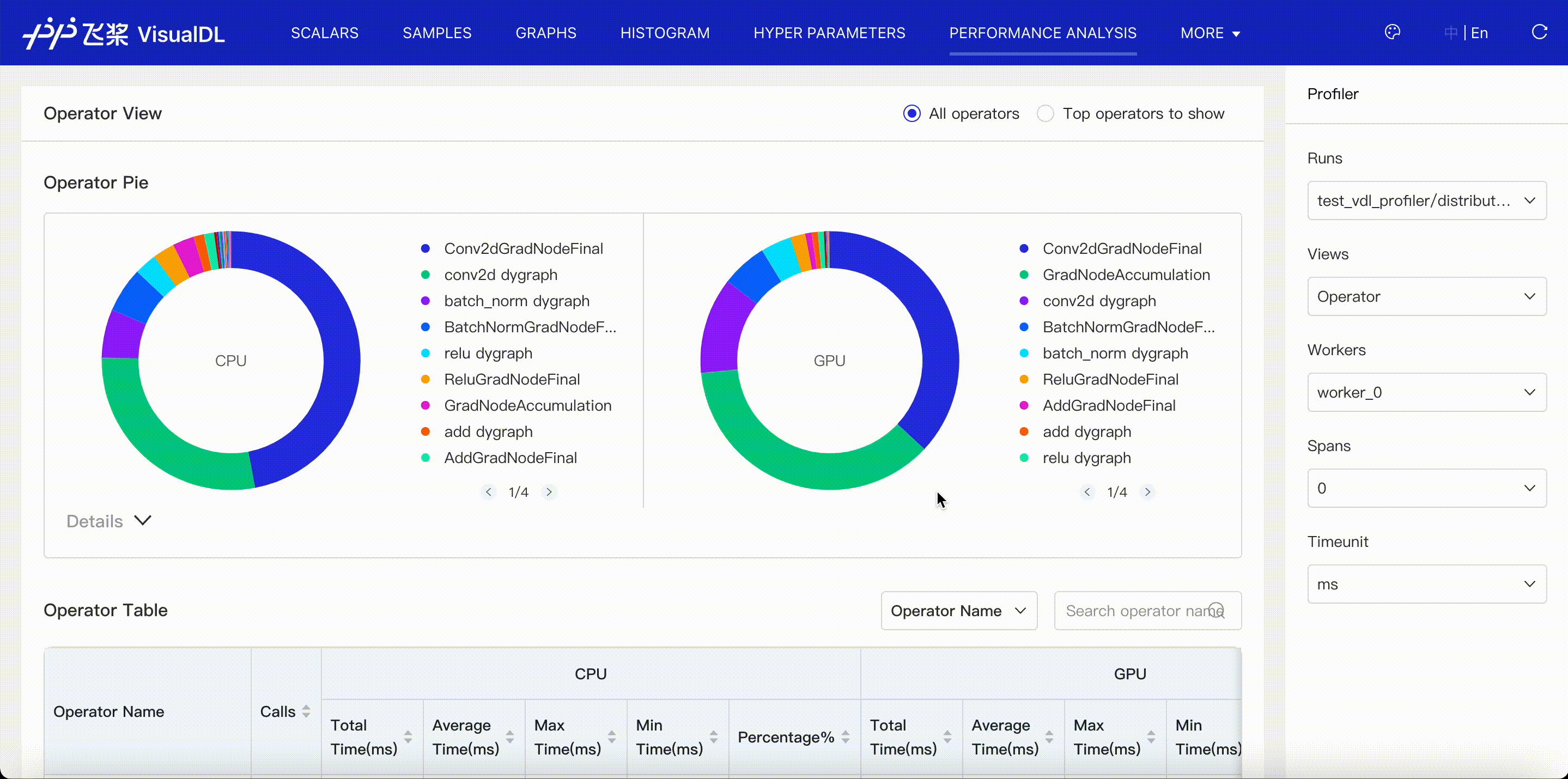## GPU Kernel¶

GPU Kernel viewer shows execution(GPU) time of kernels launched by operators. When you choose Kernel Name + Attibutes to present the kernel table, you can see kernel's corresponding operator, grid, etc.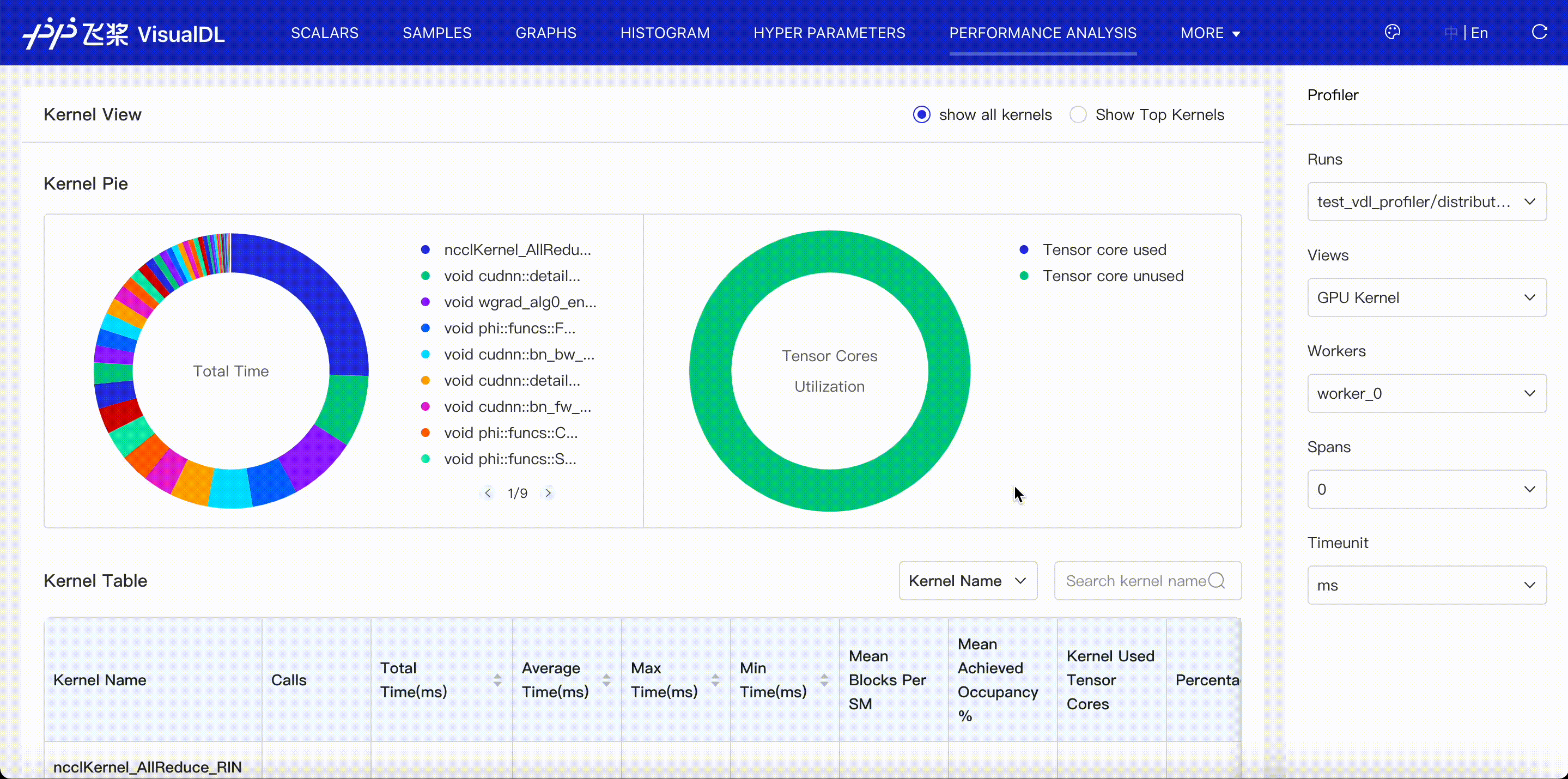## Distributed¶

Distributed viewer shows communication time, computation time and their overlap in distributed program.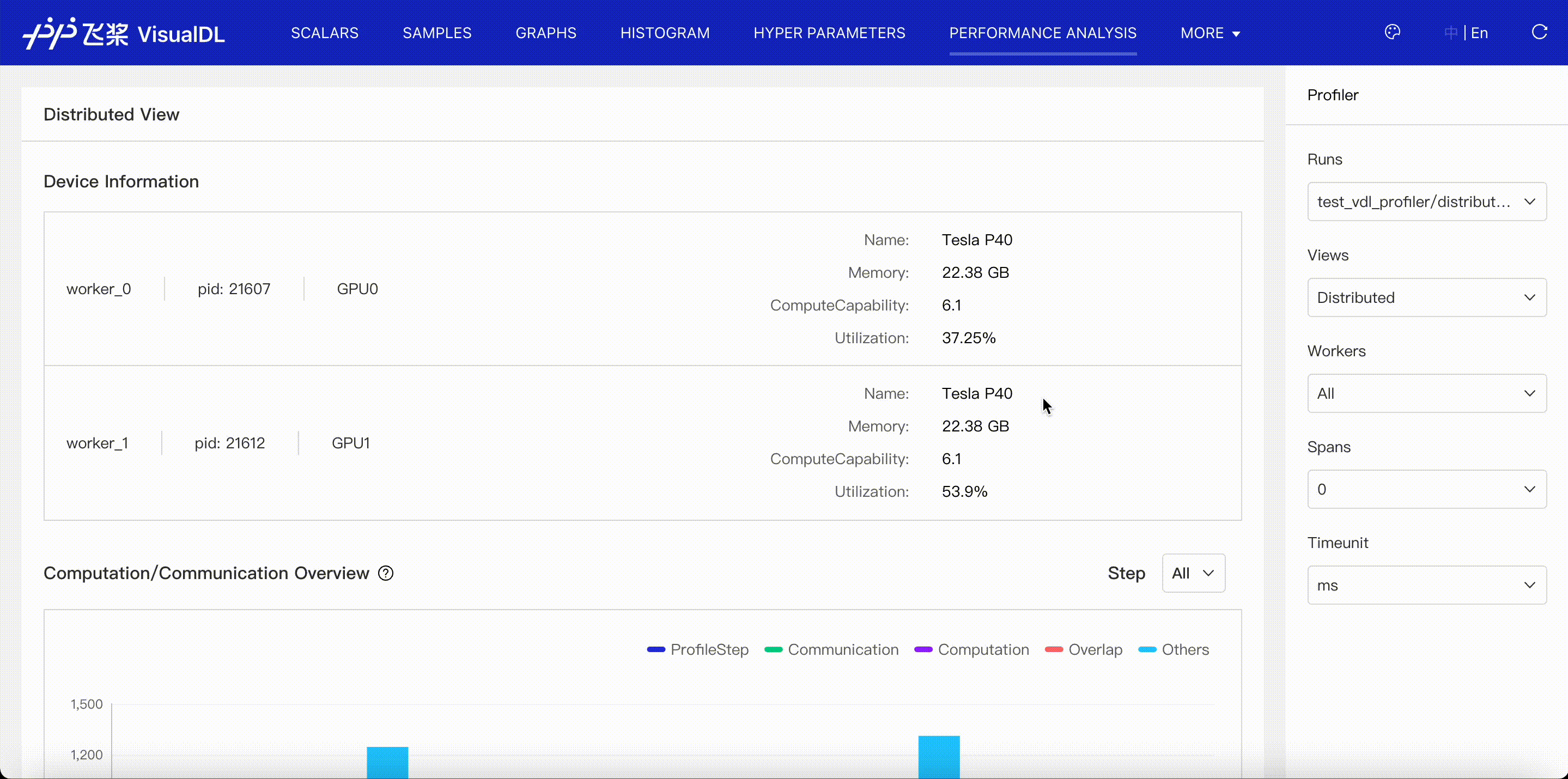• Communication: denote the time related to communication, including events of communication type in paddle framework、communication-related operators and GPU Kernels(nccl).

• Computation: denote the computation time of GPU Kernels，except communication-related Kernels(nccl).

• Overlap: denote the overlap time between Communication and Computation when they are executed parallelly.

• Others: denote the time out of Communication and Computation.

## Trace¶

Trace viewer shows the timeline of all events collected in profiling data.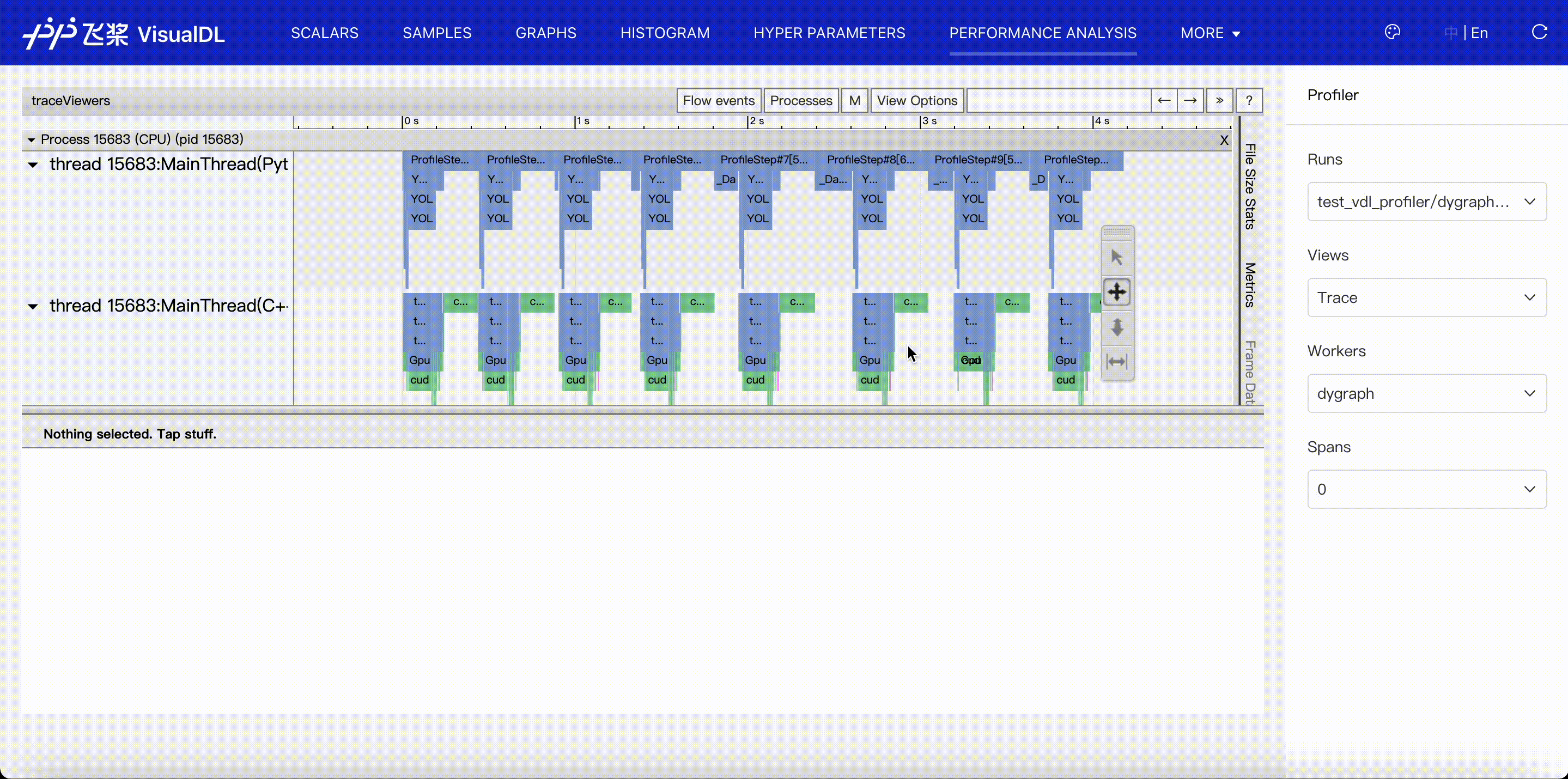• Events are listed in different threads or streams by time order, and split into Python and C++ group according to where they are recorded, so it's convenient to fold or unfold a event group. Besides, we mark the thread name if it has a name.

• Details of events are listed in the status panel. The keyboard 'w' and 's' can zoom in and zoom out the timeline, 'a' and 'd' move the timeline left and right.

• For events on GPU, you can click `launch` link to find its correlated events on CPU.

## Memory¶

Memory viewer shows the events of memory allocation and release.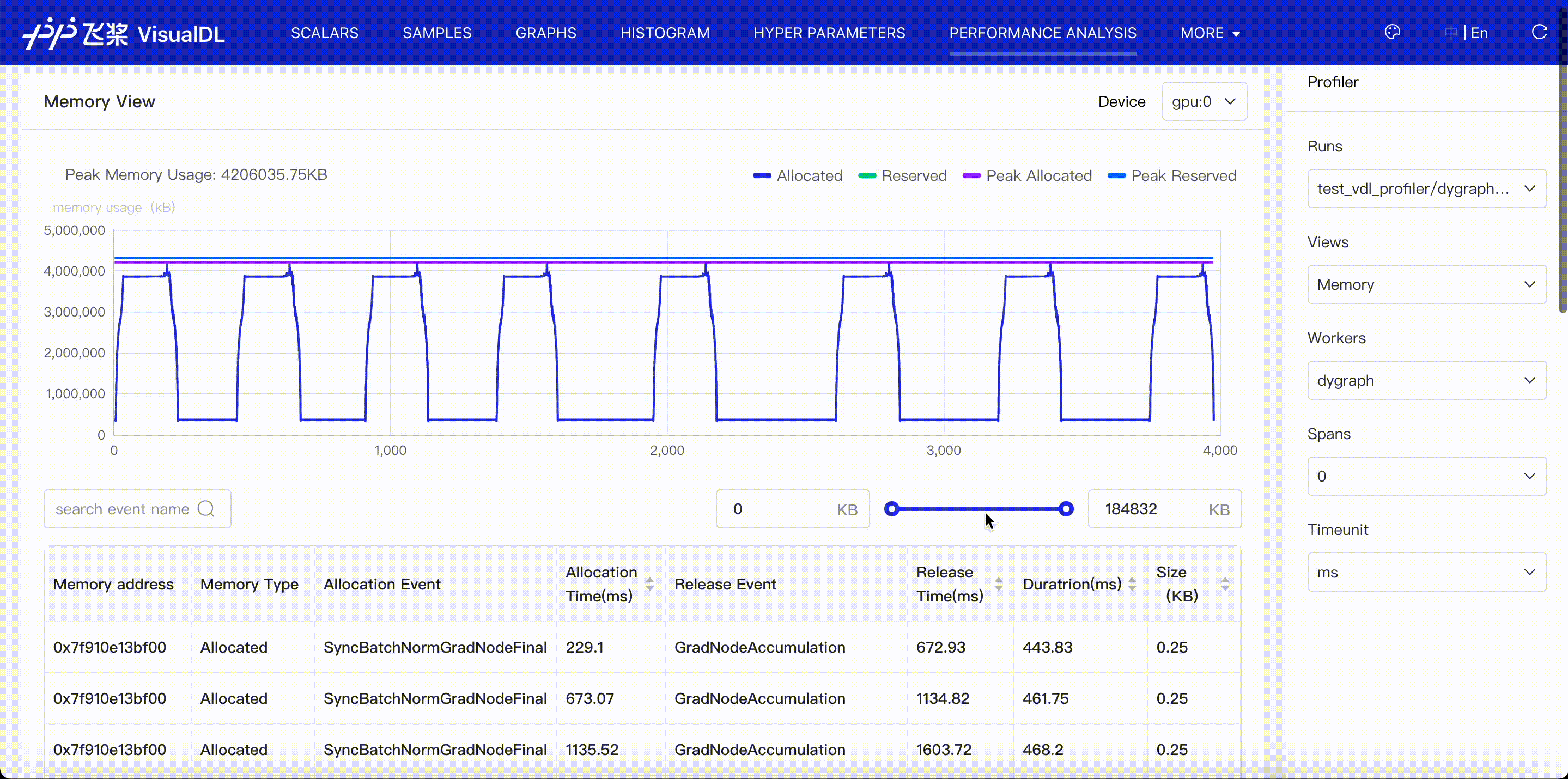Memory management is scheduled by paddle. First, paddle will apply for a large memory region as cache, this is called reserved memory. Then paddle allocates some memory for tensor creation when needed, and this is called allocated memory.

• Present the memory curve of allocated and reserved memory.

• Present the allocation and release time and events for each memory address.

• Present the allocation and release count and size for each event, and the increased memory size at the end of profiling period.